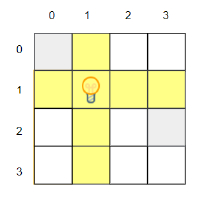# C++ program to find out the maximum number of cells that can be illuminated

Suppose, we are given a grid of dimensions h * w. The cells in the grid can contain either a bulb or obstacles. A light bulb cell illuminates the cells in its right, left, up, and down and the light can shine through the cells unless an obstacle cell blocks the light. An obstacle cell can not be illuminated and it blocks the light from a bulb cell from reaching the other cells. We are given the grid in an array of strings, where '#' represents an obstacle and '.' represents a vacant cell. We have only one bulb and we have to find out the maximum number of cells that we can illuminate placing the bulb optimally in the grid.

So, if the input is like h = 4, w = 4, grid = {"#...", "....", "...#", "...."}, then the output will be 7.From the image, we can see the illuminated cells in the grid.

## Steps

To solve this, we will follow these steps −

Define one 2D array first
for initialize i := 0, when i < h, update (increase i by 1), do:
count := 0
for initialize j := 0, when j < w, update (increase j by 1), do:
if grid[i, j] is same as '#', then:
count := 0
else:
first[i, j] := count
(increase count by 1)
k := 0
for initialize j := w - 1, when j >= 0, update (decrease j by 1), do:
if grid[i, j] is same as '#', then:
k := 0
else:
k := maximum of k and first[i, j]
first[i, j] := k
Define one 2D array second
for initialize j := 0, when j < w, update (increase j by 1), do:
count := 0
for initialize i := 0, when i < h, update (increase i by 1), do:
if grid[i, j] is same as '#', then:
count := 0
else:
second[i, j] := count
(increase count by 1)
k := 0
for initialize i := h - 1, when i >= 0, update (decrease i by 1), do:
if grid[i, j] is same as '#', then:
k := 0
else:
k := maximum of k and second[i, j]
second[i, j] := k
result := 0
for initialize i := 0, when i < h, update (increase i by 1), do:
for initialize j := 0, when j < w, update (increase j by 1), do:
result := maximum of result and first[i, j] + second[i, j]
return result + 1

## Example

Let us see the following implementation to get better understanding −

#include <bits/stdc++.h>
using namespace std;

int solve(int h, int w, vector<string> grid){
vector<vector<int>> first(h, vector<int> (w));
for(int i = 0; i < h; i++) {
int count = 0;
for(int j = 0; j < w; j++) {
if(grid[i][j] == '#') {
count = 0;
continue;
} else {
first[i][j] = count;
count++;
}
}
int k = 0;
for(int j = w-1; j >= 0; j--) {
if(grid[i][j] == '#') {
k = 0;
continue;
} else {
k = max(k, first[i][j]);
first[i][j] = k;
}
}
}
vector<vector<int>> second(h, vector<int> (w));
for(int j = 0; j < w; j++) {
int count = 0;
for(int i = 0; i < h; i++) {
if(grid[i][j] == '#') {
count = 0;
continue;
} else {
second[i][j] = count;
count++;
}
}
int k = 0;
for(int i = h-1; i >= 0; i--) {
if(grid[i][j] == '#') {
k = 0;
continue;
} else {
k = max(k, second[i][j]);
second[i][j] = k;
}
}
}
int result = 0;
for(int i = 0; i < h; i++) {
for(int j = 0; j < w; j++) {
result = max(result, first[i][j] + second[i][j]);
}
}
return result + 1;
}
int main() {
int h = 4, w = 4;
vector<string> grid = {"#...", "....", "...#", "...."};
cout<< solve(h, w, grid);
return 0;
}

## Input

4, 4, {"#...", "....", "...#", "...."}

## Output

7